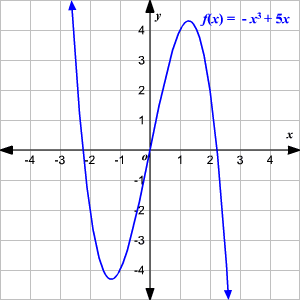The graph of the polynomial function $f\left(x\right)={a}_{n}{x}^{n}+{a}_{n-1}{x}^{n-1}+...+{a}_{1}x+{a}_{0}$ eventually rises or falls depends on the leading coefficient $\left({a}_{n}\right)$ and the degree of the polynomial function.

 Case End Behavior of graph When $n$ is odd and ${a}_{n}$ is positive Graph falls to the left and rises to the right When $n$ is odd and ${a}_{n}$ is negative Graph rises to the left and falls to the right When $n$ is even and ${a}_{n}$ is positive Graph rises to the left and right When $n$ is even and ${a}_{n}$ is negative Graph falls to the left and right

Example:

Use the Leading Coefficient Test to determine the end behavior of the graph of the polynomial function $f\left(x\right)=-{x}^{3}+5x$ .

Solution:

Because the degree is odd and the leading coefficient is negative, the graph rises to the left and falls to the right as shown in the figure.